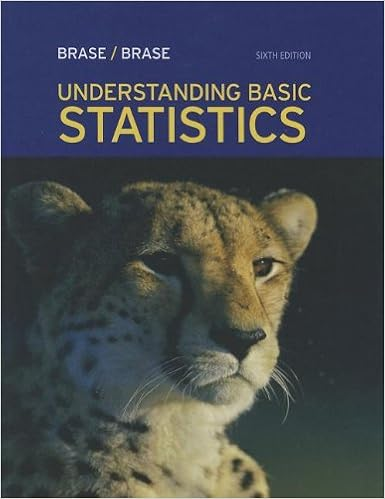# Overview two graphs that can be used to summarize the

• Homework Help
• 93
• 67% (3) 2 out of 3 people found this document helpful

This preview shows page 19 - 20 out of 93 pages.

##### We have textbook solutions for you!
The document you are viewing contains questions related to this textbook.The document you are viewing contains questions related to this textbook.
Chapter 10 / Exercise 14
Understanding Basic Statistics
Brase/BraseExpert Verified
Overview:Two graphs that can be used to summarize the distribution for a single quantitative variable or response are a histogramand a boxplot. Each graph provides different information about the distribution. When used properly, graphs can be a very effective way to summarize data. Data on a single quantitative variable should first be examined graphically. The overall shape of the distribution and existence of outliers can generally be used to assess if the data appear to be coming from a relatively homogenous population. If so, then various numerical summaries may be used to characterize the center of the distribution (such as mean and median) and the spread of the distribution (such as the standard deviation and the IQR). For categorical variables, a bar chartcan be used to display the number falling in each category (frequency distribution). Histograms:A histogram displays the distribution of a quantitative variable by showing the frequency (count) or percent of the values that are in various classes. The classes are typically intervals of numbers that cover the full range of the variable. Histograms can be used to assess the symmetry andmodalityof a single distribution or for comparing the relative locations and shapes of several distributions. Boxplots:One plot that can detect extreme observations or outliers is the boxplot.A boxplot is a graphical representation of the five-number summary, namely, the minimum, first quartile, median, third quartile, and maximum of the data. The centerline of the box marks the median or the 50thpercentile. The sides of the box show the first (lower) quartile, Q1, and the third (upper) quartile, Q3. Thus a boxplot shows the overall range (maximum – minimum) and the interquartile range(IQR = Q3 – Q1). A modified boxplot uses a rule for identifying values that are extraordinary compared to the others (outliersor outside values). Circles (o) are used to denote outliers and asterisks (*) to denote extreme outliers if any are present. Any point below Q1 – (1.5 x IQR) or above Q3 + (1.5 x IQR) is considered an outlier. Extreme outliers are points below Q1 – (2 x IQR) or above Q3 + (2 x IQR). Box plots cannot tell you the shape of the distribution.Side-by-side Boxplots:These plots are helpful for comparing two or more distributions with respect to the five-number summary. For example, suppose you are interested in comparing the distribution of a variable, such as the salary of the employees of a certain company. If you have information on job category, you might be interested in comparing the distribution of salary by job category. In this case, the side-by-side boxplots will be an important part of the descriptive analysis of the data set involved. Bar Charts:One way to display the number or frequency distribution for a categorical variable is with a bar chart. A bar chart shows the percentage of items that fall into each categoryor value of a categorical variable.It displays a bar for eachcategorywith the height of each bar equal to the number,
##### We have textbook solutions for you!
The document you are viewing contains questions related to this textbook.The document you are viewing contains questions related to this textbook.
Chapter 10 / Exercise 14
Understanding Basic Statistics
Brase/BraseExpert Verified
•••